# Introduction

This file illustrates the class map for visualizing classification results from a support vector machine, as well as some of the other tools in the class map package.

``````library("e1071")
``````
``````##
## Attaching package: 'e1071'
``````
``````## The following object is masked _by_ '.GlobalEnv':
##
##     sigmoid
``````
``````library("classmap")
``````

# Small toy example

The example from the paper, the Amazon book reviews data, takes a long time to run. As a simpler illustration we first run a small toy example.

The data is artificial and copied verbatim from Section 9.6.2 (page 363) of “An Introduction to Statistical Learning” by G. James, D. Witten, T. Hastie and R. Tibshirani (Springer eBook, eighth printing, 2017).

First generate the data and inspect it. We see that class 1 is disconnected, and class 2 lies in the middle. In the original space, linear separation is not possible.

``````set.seed(1)
X = matrix(rnorm(200*2),ncol=2)
X[1:100,] = X[1:100,]+2
X[101:150,] = X[101:150,]-2
y = as.factor(c(rep("blue",150),rep("red",50))) # two classes
table(y)
``````
``````## y
## blue  red
##  150   50
``````
``````dim(X) # 200 2
``````
``````##  200   2
``````
``````length(y) # 200
``````
``````##  200
``````
``````cols = c("deepskyblue3","red")
col = cols[as.numeric(y)]
plot(X,col=col,pch=19)
``````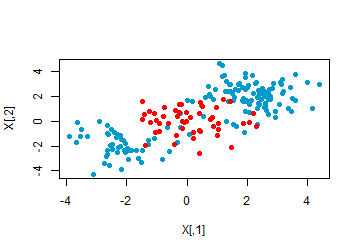We now fit an SVM with radial basis kernel to the data. We fix the seed in order to make the result of svm() reproducible:

``````dat = data.frame(X=X,y=y)
set.seed(1)
gamma=1, probability=T)
``````

Now we inspect the fitted svm. We also plot the decision values and the fit. We see that the decision value separates the classes reasonably well. The decision boundary is far from linear, but it is linear in the feature space:

``````names(svmfit)
``````
``````##   "call"            "type"            "kernel"          "cost"
##   "degree"          "gamma"           "coef0"           "nu"
##   "epsilon"         "sparse"          "scaled"          "x.scale"
##  "y.scale"         "nclasses"        "levels"          "tot.nSV"
##  "nSV"             "labels"          "SV"              "index"
##  "rho"             "compprob"        "probA"           "probB"
##  "sigma"           "coefs"           "na.action"       "fitted"
##  "decision.values" "terms"
``````
``````plot(svmfit\$decision.values,col=cols[as.numeric(y)]); abline(h=0)
``````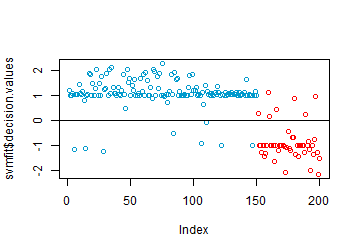``````plot(svmfit,data=dat,X.2~X.1, col = cols)
``````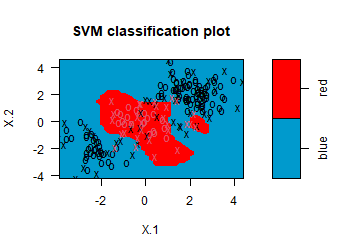Now we build the vcr object for the trained svm:

``````vcr.train = vcr.svm.train(X, y, svfit=svmfit)
``````
``````##  The kernel matrix has 4 eigenvalues below precS = 1e-12.
##  Will take this into account.
``````

We inspect the output, which contains among other things the prediction as integer, the prediction as label, the alternative label as integer and the alternative label:

``````names(vcr.train)
``````
``````##   "yint"      "y"         "levels"    "predint"   "pred"      "altint"
##   "altlab"    "PAC"       "figparams" "fig"       "farness"   "ofarness"
##  "svfit"     "X"
``````
``````vcr.train\$predint
``````
``````##    1 1 1 1 2 1 1 1 1 1 1 1 1 2 1 1 1 1 1 1 1 1 1 1 1 1 1 2 1 1 1 1 1 1 1 1 1
##   1 1 1 1 1 1 1 1 1 1 1 1 1 1 1 1 1 1 1 1 1 1 1 1 1 1 1 1 1 1 1 1 1 1 1 1 1
##   1 1 1 1 1 1 1 1 1 2 1 1 1 1 1 1 1 1 1 1 1 1 1 1 1 1 1 1 1 1 1 2 1 1 1 1 1
##  1 1 1 1 1 1 1 1 1 1 2 1 1 1 1 1 1 1 1 1 1 1 1 1 1 1 1 1 1 1 1 1 1 1 1 2 1
##  1 1 1 2 2 2 2 2 2 2 1 1 2 2 2 2 2 1 2 2 2 2 2 2 2 2 2 2 2 2 2 1 2 2 2 2 2
##  2 2 2 1 2 2 2 2 2 2 2 1 2 2 2
``````
``````vcr.train\$pred
``````
``````##    "blue" "blue" "blue" "blue" "red"  "blue" "blue" "blue" "blue" "blue"
##   "blue" "blue" "blue" "red"  "blue" "blue" "blue" "blue" "blue" "blue"
##   "blue" "blue" "blue" "blue" "blue" "blue" "blue" "red"  "blue" "blue"
##   "blue" "blue" "blue" "blue" "blue" "blue" "blue" "blue" "blue" "blue"
##   "blue" "blue" "blue" "blue" "blue" "blue" "blue" "blue" "blue" "blue"
##   "blue" "blue" "blue" "blue" "blue" "blue" "blue" "blue" "blue" "blue"
##   "blue" "blue" "blue" "blue" "blue" "blue" "blue" "blue" "blue" "blue"
##   "blue" "blue" "blue" "blue" "blue" "blue" "blue" "blue" "blue" "blue"
##   "blue" "blue" "blue" "red"  "blue" "blue" "blue" "blue" "blue" "blue"
##   "blue" "blue" "blue" "blue" "blue" "blue" "blue" "blue" "blue" "blue"
##  "blue" "blue" "blue" "blue" "blue" "red"  "blue" "blue" "blue" "blue"
##  "blue" "blue" "blue" "blue" "blue" "blue" "blue" "blue" "blue" "blue"
##  "blue" "red"  "blue" "blue" "blue" "blue" "blue" "blue" "blue" "blue"
##  "blue" "blue" "blue" "blue" "blue" "blue" "blue" "blue" "blue" "blue"
##  "blue" "blue" "blue" "blue" "blue" "blue" "red"  "blue" "blue" "blue"
##  "blue" "red"  "red"  "red"  "red"  "red"  "red"  "red"  "blue" "blue"
##  "red"  "red"  "red"  "red"  "red"  "blue" "red"  "red"  "red"  "red"
##  "red"  "red"  "red"  "red"  "red"  "red"  "red"  "red"  "red"  "blue"
##  "red"  "red"  "red"  "red"  "red"  "red"  "red"  "red"  "blue" "red"
##  "red"  "red"  "red"  "red"  "red"  "red"  "blue" "red"  "red"  "red"
``````
``````vcr.train\$altint
``````
``````##    2 2 2 2 2 2 2 2 2 2 2 2 2 2 2 2 2 2 2 2 2 2 2 2 2 2 2 2 2 2 2 2 2 2 2 2 2
##   2 2 2 2 2 2 2 2 2 2 2 2 2 2 2 2 2 2 2 2 2 2 2 2 2 2 2 2 2 2 2 2 2 2 2 2 2
##   2 2 2 2 2 2 2 2 2 2 2 2 2 2 2 2 2 2 2 2 2 2 2 2 2 2 2 2 2 2 2 2 2 2 2 2 2
##  2 2 2 2 2 2 2 2 2 2 2 2 2 2 2 2 2 2 2 2 2 2 2 2 2 2 2 2 2 2 2 2 2 2 2 2 2
##  2 2 1 1 1 1 1 1 1 1 1 1 1 1 1 1 1 1 1 1 1 1 1 1 1 1 1 1 1 1 1 1 1 1 1 1 1
##  1 1 1 1 1 1 1 1 1 1 1 1 1 1 1
``````
``````vcr.train\$altlab
``````
``````##    "red"  "red"  "red"  "red"  "red"  "red"  "red"  "red"  "red"  "red"
##   "red"  "red"  "red"  "red"  "red"  "red"  "red"  "red"  "red"  "red"
##   "red"  "red"  "red"  "red"  "red"  "red"  "red"  "red"  "red"  "red"
##   "red"  "red"  "red"  "red"  "red"  "red"  "red"  "red"  "red"  "red"
##   "red"  "red"  "red"  "red"  "red"  "red"  "red"  "red"  "red"  "red"
##   "red"  "red"  "red"  "red"  "red"  "red"  "red"  "red"  "red"  "red"
##   "red"  "red"  "red"  "red"  "red"  "red"  "red"  "red"  "red"  "red"
##   "red"  "red"  "red"  "red"  "red"  "red"  "red"  "red"  "red"  "red"
##   "red"  "red"  "red"  "red"  "red"  "red"  "red"  "red"  "red"  "red"
##   "red"  "red"  "red"  "red"  "red"  "red"  "red"  "red"  "red"  "red"
##  "red"  "red"  "red"  "red"  "red"  "red"  "red"  "red"  "red"  "red"
##  "red"  "red"  "red"  "red"  "red"  "red"  "red"  "red"  "red"  "red"
##  "red"  "red"  "red"  "red"  "red"  "red"  "red"  "red"  "red"  "red"
##  "red"  "red"  "red"  "red"  "red"  "red"  "red"  "red"  "red"  "red"
##  "red"  "red"  "red"  "red"  "red"  "red"  "red"  "red"  "red"  "red"
##  "blue" "blue" "blue" "blue" "blue" "blue" "blue" "blue" "blue" "blue"
##  "blue" "blue" "blue" "blue" "blue" "blue" "blue" "blue" "blue" "blue"
##  "blue" "blue" "blue" "blue" "blue" "blue" "blue" "blue" "blue" "blue"
##  "blue" "blue" "blue" "blue" "blue" "blue" "blue" "blue" "blue" "blue"
##  "blue" "blue" "blue" "blue" "blue" "blue" "blue" "blue" "blue" "blue"
``````

Now inspect the Probability of Alternative Class (PAC) of each object, which is one of the ingredients for the class map:

``````vcr.train\$PAC[1:3]
``````
``````##  0.09401983 0.12102387 0.12097698
``````
``````summary(vcr.train\$PAC)
``````
``````##    Min. 1st Qu.  Median    Mean 3rd Qu.    Max.
## 0.02257 0.09703 0.11846 0.18295 0.21565 0.89467
``````

The \$fig element of the output contains the distance from case i to class g. Let's look at it for the first 5 objects:

``````vcr.train\$fig[1:5,]
``````
``````##           [,1]      [,2]
## [1,] 0.2282057 0.9999972
## [2,] 0.5170902 1.0000000
## [3,] 0.5617682 0.9999999
## [4,] 0.6184622 0.9999999
## [5,] 0.8863184 0.9012566
``````

From the fig, the farness of each object can be computed. The farness of an object i is the f(i,g) to its own class:

``````vcr.train\$farness[1:5]
``````
``````##  0.2282057 0.5170902 0.5617682 0.6184622 0.8863184
``````
``````summary(vcr.train\$farness)
``````
``````##    Min. 1st Qu.  Median    Mean 3rd Qu.    Max.
## 0.01464 0.23089 0.49152 0.49674 0.76438 0.98295
``````

The “overall farness” of an object is defined as the lowest f(i,g) it has to any class g (including its own):

``````summary(vcr.train\$ofarness)
``````
``````##    Min. 1st Qu.  Median    Mean 3rd Qu.    Max.
## 0.01464 0.22740 0.48312 0.47424 0.68180 0.98295
``````

Objects with ofarness > cutoff are flagged as “outliers”. These can be included in a separate column in the confusion matrix. This confusion matrix can be computed using confmat.vcr, which also returns the accuracy. To illustrate this we choose a rather low cutoff:

``````confmat.vcr(vcr.train, cutoff = 0.975)
``````
``````##
## Confusion matrix:
##       predicted
## given  blue red outl
##   blue  140   7    3
##   red     7  43    0
##
## The accuracy is 93%.
``````

With the default cutoff = 0.99 no objects are flagged in this example:

``````confmat.vcr(vcr.train)
``````
``````##
## Confusion matrix:
##       predicted
## given  blue red
##   blue  143   7
##   red     7  43
##
## The accuracy is 93%.
``````

Note that the accuracy is computed before any objects are flagged, so it does not depend on the cutoff.

The confusion matrix can also be constructed showing class numbers instead of labels. This option can be useful for long level names.

``````confmat.vcr(vcr.train,showClassNumbers=T)
``````
``````##
## Confusion matrix:
##      predicted
## given   1   2
##     1 143   7
##     2   7  43
##
## The accuracy is 93%.
``````

A stacked mosaic plot made with the stackedplot() function can be used to visualize the confusion matrix. The outliers, if there are any, appear as grey areas on top.

``````stackedplot(vcr.train, classCols = cols, separSize = 1.5,
minSize=1, showLegend=T, cutoff = 0.975)
``````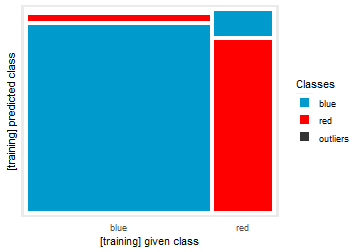``````stplot = stackedplot(vcr.train, classCols = cols,
separSize = 1.5, minSize = 1)
stackedplot(vcr.train,classCols=cols)
``````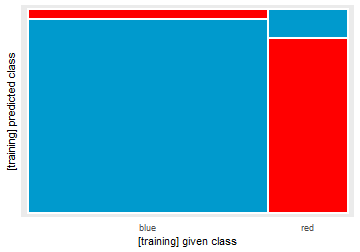We now make the class maps based on the vcr object. This can be done using the classmap() function. We make a separate class map for each of the two classes. We see that for class 1 (blue), most of the points have a low PAC, with some exceptions that are assigned to the red class. For class 2, the PAC is typically higher than in class 1.

``````par(mfrow = c(1,1))
classmap(vcr.train,1,classCols=cols)
``````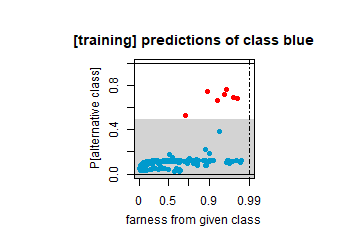``````classmap(vcr.train,2,classCols=cols)
``````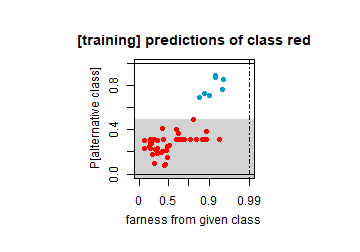``````par(oldpar)
``````

To illustrate the use of new data we create a fake dataset which is a subset of the training data, where not all classes occur, and ynew has NA's.

``````Xnew = X[c(1:25,151:175),]; dim(Xnew) # 50 200
``````
``````##  50  2
``````
``````ynew = y
ynew[c(21:25,171:175)] = NA
(ynew = ynew[c(1:25,151:175)])
``````
``````##   blue blue blue blue blue blue blue blue blue blue blue blue blue blue blue
##  blue blue blue blue blue <NA> <NA> <NA> <NA> <NA> red  red  red  red  red
##  red  red  red  red  red  red  red  red  red  red  red  red  red  red  red
##  <NA> <NA> <NA> <NA> <NA>
## Levels: blue red
``````
``````length(ynew) # 50
``````
``````##  50
``````

Now we build the vcr object on the training data.

``````vcr.test = vcr.svm.newdata(Xnew,ynew,vcr.train)
``````

Inspect some of the output in the vcr object:

``````vcr.test\$predint
``````
``````##   1 1 1 1 2 1 1 1 1 1 1 1 1 2 1 1 1 1 1 1 1 1 1 1 1 1 2 2 2 2 2 2 2 1 1 2 2 2
##  2 2 1 2 2 2 2 2 2 2 2 2
``````
``````length(vcr.test\$predint)
``````
``````##  50
``````
``````vcr.test\$altint
``````
``````##    2  2  2  2  2  2  2  2  2  2  2  2  2  2  2  2  2  2  2  2 NA NA NA NA NA
##   1  1  1  1  1  1  1  1  1  1  1  1  1  1  1  1  1  1  1  1 NA NA NA NA NA
``````
``````length(vcr.test\$altint)
``````
``````##  50
``````
``````vcr.test\$PAC
``````
``````##   0.09401983 0.12102387 0.12097698 0.11200134 0.74280537 0.11776276
##   0.11425586 0.11423242 0.06909057 0.11003569 0.11774370 0.09920899
##  0.15132460 0.72382279 0.11949166 0.11608047 0.03813805 0.04069762
##  0.11837784 0.06750460         NA         NA         NA         NA
##          NA 0.72717990 0.30206432 0.31008526 0.23122056 0.31003001
##  0.19608234 0.21809822 0.30925530 0.89467121 0.69274495 0.31001292
##  0.31009596 0.31004299 0.15351129 0.31001652 0.76492576 0.25272016
##  0.31002668 0.30996895 0.31001778         NA         NA         NA
##          NA         NA
``````
``````length(vcr.test\$PAC)
``````
``````##  50
``````
``````vcr.test\$farness
``````
``````##   0.22820570 0.51709023 0.56176824 0.61846219 0.88631840 0.60992057
##   0.17538707 0.13358437 0.04280530 0.20360078 0.49958306 0.09426870
##  0.56491943 0.95434227 0.44363884 0.54369332 0.03000818 0.35147661
##  0.17175915 0.04484612         NA         NA         NA         NA
##          NA 0.87424501 0.09672060 0.25036930 0.32597207 0.76889794
##  0.40301951 0.30435297 0.26775098 0.92350873 0.83973047 0.88157607
##  0.63707967 0.61468024 0.49043616 0.28028676 0.94811135 0.19185414
##  0.42429948 0.21034919 0.70883695         NA         NA         NA
##          NA         NA
``````
``````length(vcr.test\$farness)
``````
``````##  50
``````
``````vcr.test\$ofarness
``````
``````##   0.22820570 0.51709023 0.56176824 0.61846219 0.88631840 0.60992057
##   0.17538707 0.13358437 0.04280530 0.20360078 0.49958306 0.09426870
##  0.56491943 0.18113392 0.44363884 0.54369332 0.03000818 0.35147661
##  0.17175915 0.04484612 0.64217091 0.08903563 0.09212679 0.83534135
##  0.38530462 0.65827312 0.09672060 0.25036930 0.32597207 0.76889794
##  0.40301951 0.30435297 0.26775098 0.79915340 0.82233970 0.79379338
##  0.63707967 0.61468024 0.49043616 0.28028676 0.63481907 0.19185414
##  0.42429948 0.21034919 0.70883695 0.86225197 0.33040746 0.45583838
##  0.21736764 0.79502931
``````
``````length(vcr.test\$ofarness)
``````
``````##  50
``````

Now perform some check to confirm that it corresponds with what we would expect, given that the test data is a subset of the training data:

``````plot(vcr.test\$yintnew[c(1:20,26:45)],
vcr.train\$yint[c(1:20,151:170)]); abline(0,1)
``````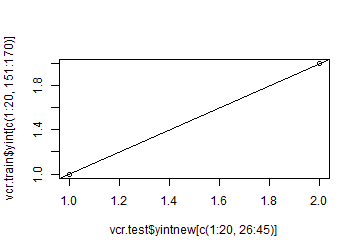``````plot(vcr.test\$predint[c(1:20,26:45)],
vcr.train\$predint[c(1:20,151:170)]); abline(0,1)
``````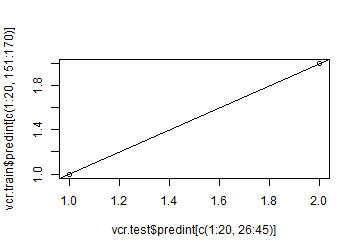``````plot(vcr.test\$altint[c(1:20,26:45)],
vcr.train\$altint[c(1:20,151:170)]); abline(0,1)
``````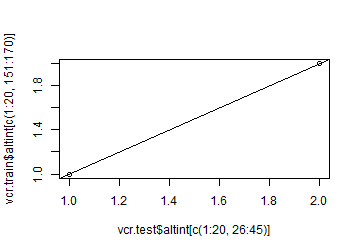``````plot(vcr.test\$PAC[c(1:20,26:45)],
vcr.train\$PAC[c(1:20,151:170)]); abline(0,1)
``````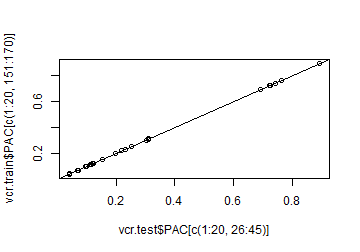``````plot(as.vector(vcr.test\$fig[c(1:20,26:45),]),
as.vector(vcr.train\$fig[c(1:20,151:170),])); abline(0,1)
``````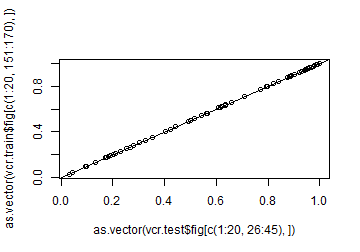``````plot(vcr.test\$farness[c(1:20,26:45)],
vcr.train\$farness[c(1:20,151:170)]); abline(0,1)
``````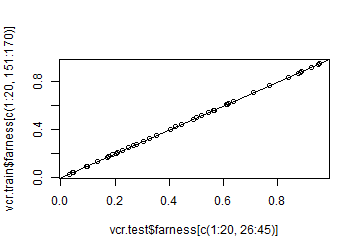``````plot(vcr.test\$ofarness[c(1:20,26:45)],
vcr.train\$ofarness[c(1:20,151:170)]); abline(0,1)
``````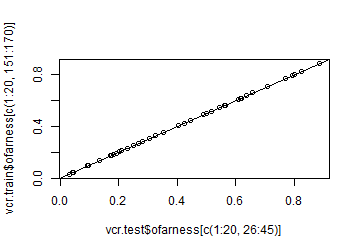Now we redo the analysis in feature space. What do these data look like in feature space? In order to answer the question, we first compute the gaussian kernel used in svmfit:

``````Kxx = makeKernel(X,svfit=svmfit)
dim(Kxx)
``````
``````##  200 200
``````

Now we can compute a representation of these points in feature space. The function MakeFV() takes a kernel, and from it creates a feature space with the desired inner products. The kernel matrix has a few eigenvalues below the precision precS, and this is taken into account:

``````outFV = makeFV(Kxx)
``````
``````##  The kernel matrix has 4 eigenvalues below precS = 1e-12.
##  Will take this into account.
``````
``````Xf = outFV\$Xf
``````

As a sanity check, we make sure that the kernel matrix and the inner products of Xf match (almost exactly):

``````max(abs(as.vector(Kxx - Xf%*%t(Xf)))) # tiny, this is good.
``````
``````##  6.167711e-11
``````

The rows of Xf are in 196-dimensional space, do we need all of those dimensions? It turns out that we do need many. More precisely, we need 39 components to explain 95% of the variance. That's many when starting from bivariate data.

``````explvar = cumsum(apply(Xf,2,var))
plot(explvar/explvar[ncol(Xf)]); abline(h=0.95)
``````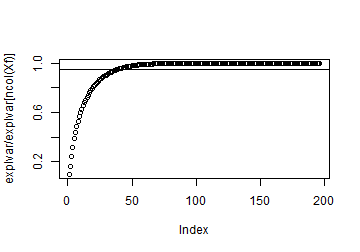``````min(which(explvar > 0.95*explvar[ncol(Xf)]))
``````
``````##  39
``````

We inspect the data in feature space a little bit more. We see that all points in Xf lie on the unit sphere. This is always true for the radial basis kernel.

``````range(rowSums(Xf^2))
``````
``````##  1 1
``````

In some of the pairwise plots, we can also see spherical effects. The data does look more separable here than in the original two-dimensional space.

``````pairs(Xf[,1:5], col=col)
``````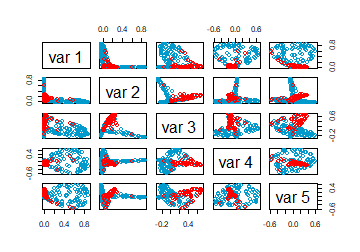``````plot(Xf[,1],Xf[,5],col=col,pch=19)
``````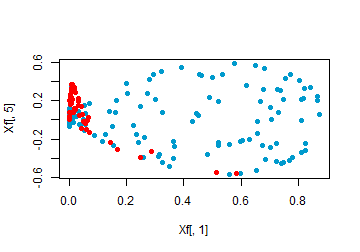Now we run SVM on Xf with linear kernel and the same cost. This yields the same result as above:

``````Xfdat = data.frame(X=Xf,y=y)
set.seed(1)
svmfit1 = svm(y~.,data=Xfdat,scale=F,kernel="linear",cost=10,
probability=T)
plot(svmfit1\$decision.values,svmfit\$decision.values); abline(0,1)
``````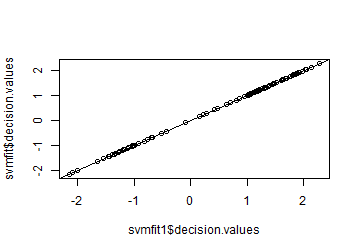``````vcr.trainf = vcr.svm.train(X=Xf, y, svfit=svmfit1)
``````

We see that everything matches with the original svm which was trained using a gaussian kernel on the original data:

``````plot(vcr.train\$yint,vcr.trainf\$yint); abline(0,1)
``````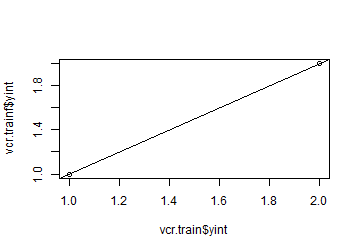``````plot(vcr.train\$predint,vcr.trainf\$predint); abline(0,1)
``````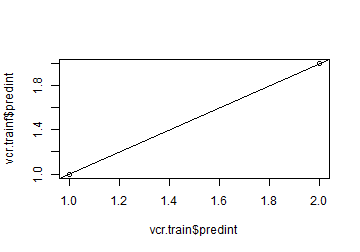``````plot(vcr.train\$altint,vcr.trainf\$altint); abline(0,1)
``````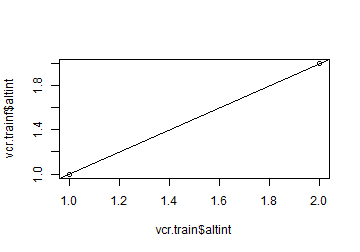``````plot(vcr.train\$PAC,vcr.trainf\$PAC); abline(0,1)
``````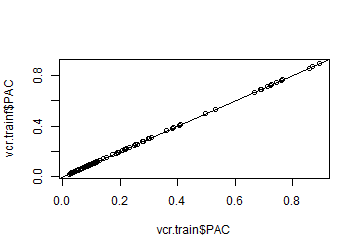``````plot(vcr.train\$fig,vcr.trainf\$fig); abline(0,1)
``````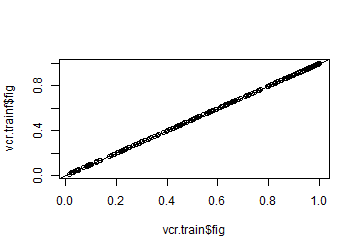``````vcr.train\$figparams\$farscales
``````
``````##  1 1
``````
``````plot(vcr.train\$farness,vcr.trainf\$farness); abline(0,1)
``````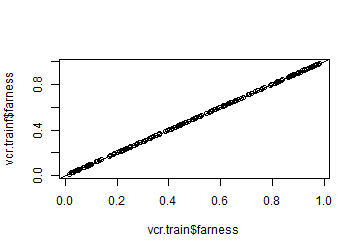``````plot(vcr.train\$ofarness,vcr.trainf\$ofarness); abline(0,1)
``````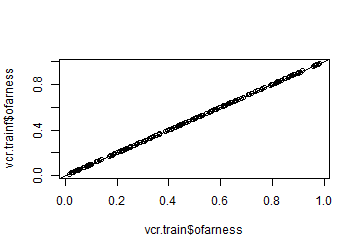We now make a test data with NA's in ynew, but in feature space:

``````Xnew = Xf[c(1:25,151:175),]; dim(Xnew)
``````
``````##   50 196
``````

Using this test data, we build a new vcr object:

``````vcr.testf = vcr.svm.newdata(Xnew,ynew,vcr.trainf)
``````

Again, we see an exact match with original version:

``````plot(vcr.testf\$yintnew,vcr.test\$yintnew); abline(0,1)
``````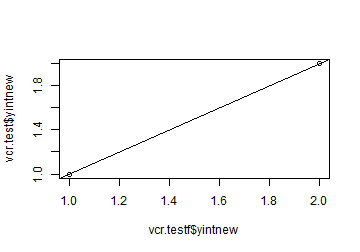``````plot(vcr.testf\$predint,vcr.test\$predint); abline(0,1)
``````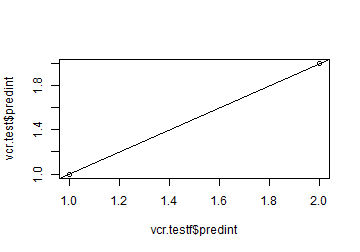``````plot(vcr.testf\$altint,vcr.test\$altint); abline(0,1)
``````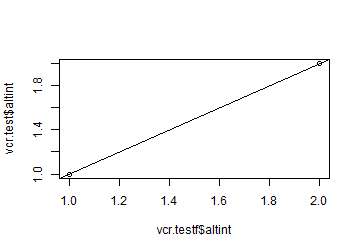``````plot(vcr.testf\$PAC,vcr.test\$PAC); abline(0,1)
``````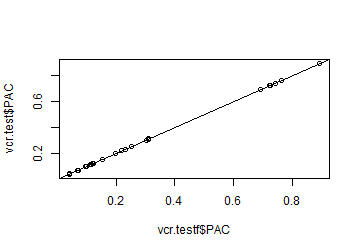``````plot(vcr.testf\$fig,vcr.test\$fig); abline(0,1)
``````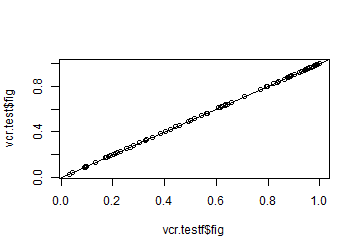``````plot(vcr.testf\$farness,vcr.test\$farness); abline(0,1)
``````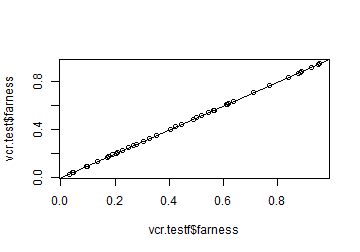# Amazon book review data

``````data(data_bookReviews)
X = data_bookReviews[1:500, 1]; y = data_bookReviews[1:500, 2]
Xnew  = data_bookReviews[501:1000, 1]; ynew = data_bookReviews[501:1000, 2]
length(X); length(Xnew); length(y); length(ynew)
``````
``````##  500
``````
``````##  500
``````
``````##  500
``````
``````##  500
``````

This is a subset of the data used in the paper, which was assembled by Prettenhofer and Stein (2010), see https://arxiv.org/abs/1008.0716

The full dataset has been used for a variety of things, including classification using svm. A list of citations: https://scholar.google.com/scholar?hl=en&as_sdt=0%2C5& sciodt=0%2C5&cites=4738650459241064524&scipsc=1&q=svm+&btnG=

The subset was chosen small enough to keep the computation time low, while still containing the examples in the paper.

First we define the colors and inspect an example of a book review:

``````cols = c("deepskyblue3","red")
col = cols[as.numeric(y)]
X
``````
``````##  "the others in the series were great, and i really looked forward to a breath of snow and ashes. it read like it was all gabaldon's discarded notes for the previous installment. i felt really cheated to have spent my time and money on this book. "
``````

The next question is what kernel to use. There are many string kernels. kernlab provides several options: The “spectrum” kernel matches substrings of an exact size. The “boundrange” kernel matches substrings of a length up to a given size. The “constant” kernel matches all substrings. The “exponential” kernel matches all substrings but gives different weights depending on the length of the matched string.

The best kernel for these data in terms of accuracy turned out to be the spectrum kernel with length 7:

``````strdot = kernlab::stringdot(type = "spectrum", length = 7)
strdot
``````
``````## String kernel function.  Type =  spectrum
##  Hyperparameters : sub-sequence/string length =  7
##  Normalized
``````
``````ptm = proc.time()
Kr_train = kernlab::kernelMatrix(kernel=strdot, x=X)
(proc.time()-ptm)
``````
``````## elapsed
##   20.89
``````
``````dim(Kr_train)
``````
``````##  500 500
``````

Using the makeFV() function, we can compute the corresponding points in feature space. It takes into account almost-zero eigenvalues. The resulting Xf can be seen as the scores of a kernel PCA without centering (mean subtraction).

``````outFV = makeFV(Kr_train)
``````
``````##  The kernel matrix has 1 eigenvalues below precS = 1e-12.
##  Will take this into account.
``````
``````Xf = outFV\$Xf
dim(Xf)
``````
``````##  500 499
``````

To see how well these feature vectors and Kr_train match, we can compute the inner products in Xf, and verify that they are close to Kr_train:

``````inprod = Xf%*%t(Xf)
max(abs(as.vector(Kr_train-inprod)))
``````
``````##  6.008671e-11
``````

We can also verify that the points of Xf lie on the unit sphere in 500 dimensions:

``````range(rowSums(Xf^2)) # all 1
``````
``````##  1 1
``````

By construction, the variables of Xf are uncorrelated. In order to explain 95% of the variance in the data, we need 463 out of the 500 components, so we are in a truly high dimensional situation:

``````explvar = cumsum(apply(Xf,2,var))
plot(explvar/explvar[ncol(Xf)]); abline(h=0.95)
``````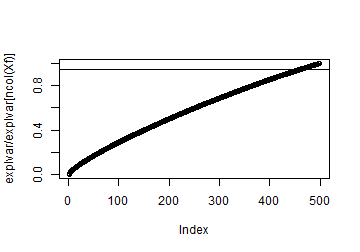``````min(which(explvar > 0.95*explvar[ncol(Xf)]))
``````
``````##  463
``````

Now we can train the support vector classifier on these data. On Xf we train the SVM with the LINEAR kernel, since we are in feature space:

``````Xfdat = data.frame(X = Xf, y = y)
set.seed(1) # we have to fix the seed!
svmfit = svm(y~.,data=Xfdat,scale=F,kernel="linear",cost=2,
probability=T)
``````

We plot the decision values. the two horizontal lines contain the support vectors. There are a lot of them, since the dimension is high.

``````plot(svmfit\$decision.values)
``````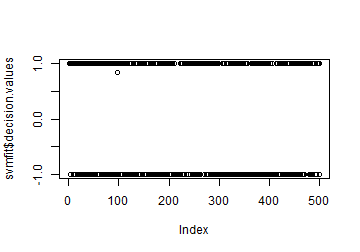Now create the VCR object of the training data:

``````vcr.train = vcr.svm.train(Xf, y, svfit=svmfit)
``````

Using the VCR object, we can build the confusion matrix. We see that the accuracy is 100%. This classification is perfect due to overfitting!

``````confmat.vcr(vcr.train, showOutliers = FALSE)
``````
``````##
## Confusion matrix:
##      predicted
## given   1   2
##     1 250   0
##     2   0 250
##
## The accuracy is 100%.
``````

The classification on the test data is more interesting. First construct the kernel matrix we need to classify the test data, which takes roughly a minute:

``````ptm = proc.time()
Kr_test = kernlab::kernelMatrix(kernel=strdot, x=Xnew, y=X)
(proc.time()-ptm)
``````
``````## elapsed
##   42.41
``````

Now we compute feature vectors of the test set. These features live in the same space as the Xf of the training data.

``````FVtest = makeFV(kmat=Kr_test, transfmat=outFV\$transfmat)
Zf = FVtest\$Xf
dim(Zf)
``````
``````##  500 499
``````

We compare the inner products with the kernel matrix to make sure that they are the same:

``````inprod = Zf%*%t(Xf)
max(abs(as.vector(Kr_test-inprod))) # tiny, good.
``````
``````##  1.15278e-11
``````

Now create the VCR object of the test data:

``````vcr.test = vcr.svm.newdata(Zf,ynew,vcr.train)
``````

Compute the confusion matrix from the VCR object. In the full dataset, the accuracy was 82 %. Here we achieve roughly 74% accuracy, which is lower since training was done on 500 texts instead of 2000.

``````confmat.vcr(vcr.test)
``````
``````##
## Confusion matrix:
##      predicted
## given   1   2
##     1 178  72
##     2  56 194
##
## The accuracy is 74.4%.
``````

The stacked mosaic plot of the classification is presented below:

``````stackedplot(vcr.test,classCols=cols,separSize=1.5,minSize=1.5)
``````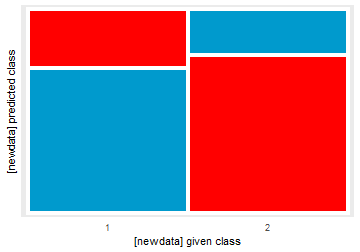Now we build the class maps for the test set. First the class map of the negative reviews:

``````# To identify the atypical points, uncomment the next line:
# classmap(vcr.test,1,classCols=cols,identify=T)
# Identified point(s):
#   67 127 118 145  94  45
#
# pdf("Bookreviews_500_classmap_test_negative.pdf")
par(mar = c(3.5, 3.5, 2.0, 0.2))
coords = classmap(vcr.test, 1, classCols = cols, cex = 1.5,
main = "predictions of the negative book reviews",
cex.lab = 1.5, cex.axis = 1.5, cex.main = 1.5,
maxfactor = 1.05, identify = FALSE)
indstomark = c(67, 127, 118, 145, 94, 45) # from identify=T above
labs  = c(  "a",  "b", "x",  "c",   "d",  "e")
xvals = coords[indstomark, 1] +
c(-0.08, 0, 0, 0.09, 0.09, 0.09) # visual fine tuning
yvals = coords[indstomark, 2] +
c(0, 0.033, 0.030, 0, 0, 0)
text(x = xvals, y = yvals, labels = labs, cex = 1.5)
legend("topright", fill = cols,
legend = c("negative", "positive"), cex = 1.4,
ncol = 1, bg = "white")
``````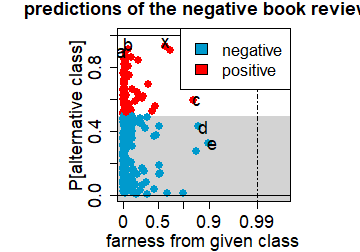``````# dev.off()
par(oldpar)
``````

We marked the following points:

``````(Xnew[which(ynew == 1)]) # (a) in the paper.
``````
``````##  "this collection consists mostly of the last stories in the past through tomorrow. ptt may well be one of heinein's masterworks, a collection of short stories that retells history from the late 1890's but puts the world on a different course. by lopping off the last few short stories, this collection looses the continuity that made the ptt a great read. . "
``````
``````(Xnew[which(ynew == 1)]) # (b) in the paper.
``````
``````##  "basically this book is an elaborate defense of promiscuity and hedonism. i have liked his other books in the past (skinny legs and all is one of my all-time favorites) but this one didn't have enough insight to adequately place the elements of the story within a greater understanding of the world around us (usually one the greatest things about his writing."
``````
``````(Xnew[which(ynew == 1)]) # (x)
``````
``````##  "bought this book as package recommended by amazon. it is not for adobe photoshop album 2.0. many changes have been made to album 2.0 and this book has many inconsistencies not usable in the new program. i highly recommend michael slater's book on album 2.0-it is up to date and useful. i returned this book today"
``````
``````# Negative review, but highly recommends another book!

(Xnew[which(ynew == 1)]) # (c) in the paper.
``````
``````##  "the cover states the book is for 'students and nonspecialist', which i found to be not true. the best example was in the chapter metaphysics, if you do not already understand the concepts and especially aquinas` arguements before reading the book you will definitly not understand them after reading the book. i felt the book confuses much more than enlightens"
``````
``````# Contains some grammatical errors
# Is negative, but expressed very indirectly.

(Xnew[which(ynew == 1)]) # (d) in the paper.
``````
``````##  "not at all as interesting as i hoped it would be. the back roads of usa should be able to offer a much more colourful and interesting web than this"
``````
``````# Definitely negative, but quite short.

(Xnew[which(ynew == 1)]) # (e) in the paper.
``````
``````##  "i have read history books more interesting than this book. when i purchased the book i thought that it would be an interesting work. the book started off interesting. then, as it progressed it got worse. rent the movie. it would be much better. trust me"
``````

Now the class map of the positive reviews in the test set:

``````#
# To identify the atypical points:
# classmap(vcr.test,2,classCols=cols,identify=T)
# Identified point(s):
#  48  32 18 168 113
#
# pdf("Bookreviews_500_classmap_test_positive.pdf")
par(mar = c(3.5, 3.5, 2.0, 0.2))
coords = classmap(vcr.test, 2, classCols = cols, cex = 1.5,
main = "predictions of the positive book reviews",
cex.lab = 1.5, cex.axis = 1.5, cex.main = 1.5,
maxfactor = 1.05, identify = FALSE)
indstomark = c(48, 32, 18, 168, 113) # from identify=T above
coords[indstomark,]
``````
``````##        qfarness       PAC
## 107 0.004741691 0.8821470
## 81  1.371590838 0.8842944
## 51  1.892822909 0.9689219
## 339 2.932466734 0.8642608
## 233 2.567124310 0.3484653
``````
``````labs  = c( "f",   "g",  "h",  "i",  "j")
xvals = coords[indstomark, 1] +
c(0, 0, 0.09, 0.09, 0.09) # visual fine tuning
yvals = coords[indstomark, 2] +
c(0.032, 0.032, 0, 0, 0)
text(x = xvals, y = yvals, labels = labs, cex = 1.5)
legend("left", fill = cols,
legend = c("negative", "positive"), cex = 1.4,
ncol = 1, bg = "white")
``````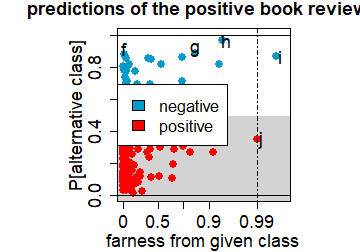``````# dev.off()
par(oldpar)
``````

We marked the following points:

``````(Xnew[which(ynew == 2)]) # (f) in the paper.
``````
``````##  "i used the first edition of stroustrip's the c++ programming language until it was dog-eared and the binding fell apart. i'm glad that this edition is hard-backed so i will not wear it out. c++ is a complex language and it's impossible to write a program of any size in c++ without a good reference on your desk. i have not found any other c++ reference that is as complete or as useful as this book. this is not an introduction to c++ but rather a reference book. i learned c++ from lippman's c++ primer but that's on my bookshelf and stroustrip is on my desk. "
``````
``````(Xnew[which(ynew == 2)]) # (g) in the paper.
``````
``````##  "to surmise, the book is about an actor who is hired to be a political figure. i will not reveal any more as to not ruin the nature of the plot. i felt that like some of heinlein's books, the book is plauged with politics, less science fiction, the only sci-fi thing about it is that the plot takes place in space, other than that, it's all politics. same thing with citizen of the galaxy, a book that starts off really well and ending with a theme that do not portray sci-fi rather it shows heinlein's knowledge in law. i felt that the book could have been much better and i do not see how it won the hugo award. conclusion: the book is a fun pass, but nothing more. do not expect any groundbreaking plot, or jaw dropping plot twists, just your old fashioned politicians-dear-diary"
``````
``````(Xnew[which(ynew == 2)]) # (h) in the paper.
``````
``````##  "this book is not for everyone. if you do not like to read classics with everything that goes along with that (such as long-winded language that seems outdated to us today), then you should stay away. for lovers of classical and relatively easy to read literature, this is a good book. a lot of things are amazing, considering how long ago they were written and what overall level of scientific knowledge was at the time. some of it just boggles my mind. at the same time, the book is long winded and in the end, not quite as much happens as one would expect. dan brown's davinci code has more things happening in the first 20 pages than verne has in his entire book. but that is ok in a way, because when i read a classic, i do not expect to compare it to modern standards. the entertainment is due to different factors. in fact, the way the book is written is part of the entertainment and not just the story. i do not give this book 5 stars however, because i was disappointed in the end, since not enough of the story really comes to a conclusion. i do not want to spoil the book for you, but there are a lot of unanswered questions, and getting those answers really was what kept me reading. there is quite a bit of build-up, and then in some ways, the book just ends. (i noticed that style in many books of that time, where the narrator just says 'here is what i know... but i do not know the whole story...'). also, to some extent, i agree with some of my fellow reviewers that gave a lower score, in that it is too much of a narration rather than a real story. i do not complain about some of it being like a log book, but i would have wished to get a bit more information about the daily life on board. i just do not buy that the 3 travelers just stayed in their room. they must have found out a bit more about other parts of the boat. or at least attempted it, and that would have been interesting to read about, without breaking with the overall style of the book"
``````
``````(Xnew[which(ynew == 2)]) # (i) in the paper.
``````
``````##  "i really loved the book i just wish the author of the book would of used kyles origin from the comics"
``````
``````(Xnew[which(ynew == 2)]) # (j) in the paper.
``````
``````##  "like the book very muc"
``````
``````# very short (and spelling error).
``````